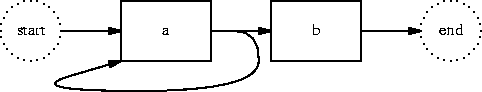Next Regular Expression Mastery 27

# Anti-Greed

• What's the opposite of `greedy'? (`Monastic'?)

• a+?b is just like a+b

• Except it tries the arrows in the other order

/a+?b/• aaab

```             START             |a a a b
a                 |a a a b         Yes!
+?                <a|a a b
b                <a|a a b         Nope
a                <a|a a b         Yes!
+?               <a a|a b
b               <a a|a b         Nope
a               <a a|a b         Yes!
+?              <a a a|b
b              <a a a|b         Yes!
END            <a a a b>        Yes!

```
• Notice more backtracking

• Usually less efficient

• That's why the `normal' one is greedy

 NextCopyright © 2002 M. J. Dominus